Courses

# Fluid Dynamics & Flow Measurements Civil Engineering (CE) Notes | EduRev

## Topic wise GATE Past Year Papers for Civil Engineering

Created by: Gate Gurus

## Civil Engineering (CE) : Fluid Dynamics & Flow Measurements Civil Engineering (CE) Notes | EduRev

The document Fluid Dynamics & Flow Measurements Civil Engineering (CE) Notes | EduRev is a part of the Civil Engineering (CE) Course Topic wise GATE Past Year Papers for Civil Engineering.
All you need of Civil Engineering (CE) at this link: Civil Engineering (CE)

Question 1. A circular duct carrying water gradually contracts from a diameter of 30 cm to 15 cm. The figure (not drawn to scale) shows the arrangement of differential manometer attached to the duct.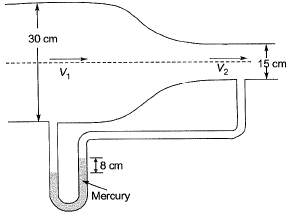When the water flows, the differential manometer shows a deflection of 8 cm of mercury (Hg). The values of specific gravity of mercury and water are 13.6 and 1.0, respectively. Consider the acceleration due to gravity, g = 9.81 m/s2. Assuming frictionless flow, the flow rate (in m3/s, round off to 3 decimal places) through the duct is _______ .
[2019 : 1 Mark, Set-I]
Solution: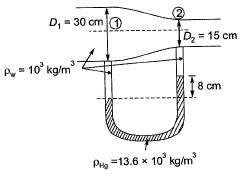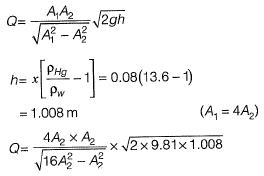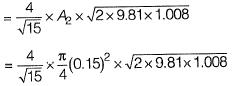Q= 0.081 m3/s (round off to 3 decimal place)

Question 2. Bernoulli’s equation is applicable for     [2018 : 1 Mark, Set-I]
(a) viscous and compressible fluid flow
(b) inviscid and compressible fluid flow
(c) inviscid and incompressible fluid flow
(d) viscous and incompressible fluid flow
Solution:

Bernoulli’s equation is application for:
1. Flow along a stream line
2. When the effect of viscosity is negligible i.e., invisid flow
3. Incompressible flow condition
Note: Even if flow is rotational, Bernoulli’s equation can be applied between two points on the same stream line

Question 3. Water flows through a 90° bend in a horizontal plane as depicted in the figure.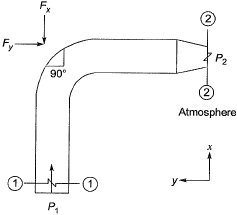A pressure of 140 kPa is measured at section 1-1. The inlet diameter marked at section 1-1 is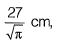while the nozzle diameter marked at section 2-2 is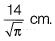Assume the following:
(i) Acceleration due to gravity = 10 m/s
(ii) Weights of both the bent pipe segment as well as water are negligible
(iii) Friction across the bend is negligible.
The magnitude of the force (in kN, up to two decimal places) that would be required to hold the pipe section is _________ .      [2017 : 2 Marks, Set-I]
Solution: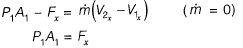Or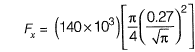=2551.5 N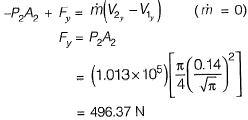Resultant force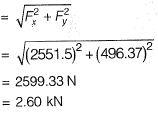Question 4.  A venturimeter having a throat diameter of 0.1 m is used to estimate the flow rate of a horizontal pipe having a diameter of 0.2 m. For an observed pressure difference of 2 m of water head and coefficient of discharge equal to unity, assuming that the energy losses are negligible, the flow rate (in m3/s) through the pipe is approximately equal to    [2014 : 2 Marks, Set-II]
(a) 0.500
(b) 0.150
(c) 0.050
(d) 0.015
(c)
Solution:

Diameter of throat,
D2 = 0.1 m
Diameter of pipe,
D1= 0.2 m
Pressure difference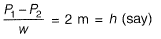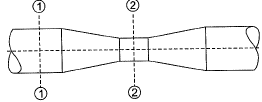Since it is given that losses are negligible. Take coefficient of discharge as,
CD= 1
Discharge,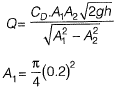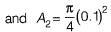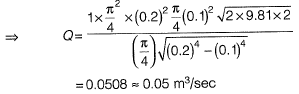Question 5.  A venturimeter, having a diameter of 7.5 cm at the throat and 15 cm at the enlarged end, is installed in a horizontal pipeline of 15 cm diameter. The pipe carries an incompressible fluid at a steady rate of 30 litres per second. The difference of pressure head measured in terms of the moving fluid in between the enlarged and the throat of the venturimeter is observed to be 2.45 m. Taking the acceleration due to gravity as 9.81 m/s2, the coefficient of discharge of the venturimeter (correct up to two places of decimal) is ____ .     [2014 : 2 Marks, Set-I]
Solution: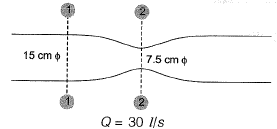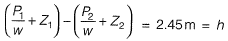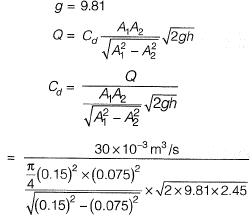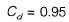Question 6. Group-I lists a few devices while Group-ll provides information about their uses. Match the devices with their corresponding use.
Group-i
P. Anemometer
Q. Hygrometer
R. Pitot Tube
S. Tensiometer
Group-ll
1. Capillary potential of soil water
2. Fluid velocity at a specific point in the flow stream
3. Water vapour content of air
4. Wind speed    [2014:1 Mark, Set-II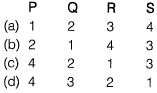(d)
Solution:
Hygrometer: A hygrometer is an instrument used for measuring the amount of humidity and water vapour in the atmosphere, in soil or in confined spaces.
Tensiometer: It is used to measure capillary potential of soil water.

Question 7. Match List-I (Devices) with List-ll (Uses) and select the answer using the codes given below the lists:
List-I
A. Pitot tube
B. Manometer
C. Venturimeter
D. Anemometer
List-ll
1. Measuring pressure in a pipe
2. Measuring velocity of flow in a pipe
3. Measuring air and gas velocity
4. Measuring discharge in a pipe
Codes:   [2010 : 1 Mark]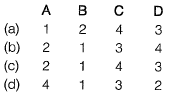(c)
Solution:
Pitot tube: It is used to measure velocity of fluid. Difference in the readings of pitot tube and piezometer (h), indicates the velocity head. This is with the assumption that pierometric head at A and C is same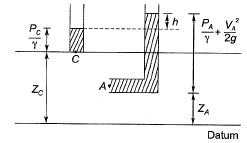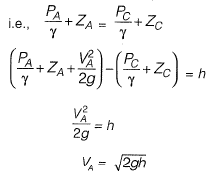Manometer: Measureiy pressure in a pipe.
Venturimeter: Measuring discharge in a pipe.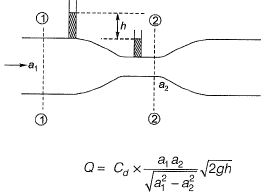Anemometer: Used to measure air velocity.

Offer running on EduRev: Apply code STAYHOME200 to get INR 200 off on our premium plan EduRev Infinity!

69 docs

,

,

,

,

,

,

,

,

,

,

,

,

,

,

,

,

,

,

,

,

,

;